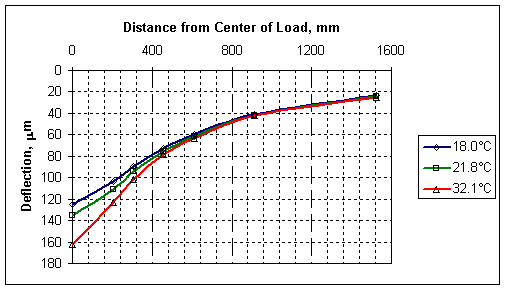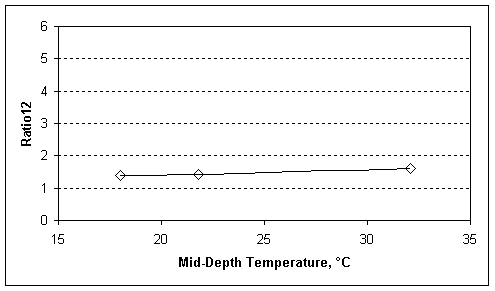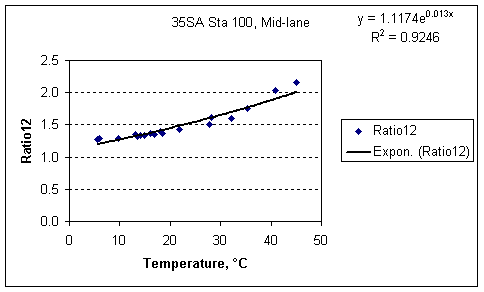U.S. Department of Transportation
1200 New Jersey Avenue, SE
Washington, DC 20590
202-366-4000

Federal Highway Administration Research and Technology
Coordinating, Developing, and Delivering Highway Transportation InnovationsThis report is an archived publication and may contain dated technical, contact, and link information
 Federal Highway Administration > Publications > Research Publications > LTPP Publications >   Ratio.Cfm The 'Ratio' Basin Shape Factors
 Publication Number: FHWA-RD-98-085 Date: November 2003

# The 'Ratio' Basin Shape Factors

Like the Delta basin shape factors, the "Ratio" basin shape factors date back to the early days of deflection testing, and are quite simple in concept. Simply put, a "Ratio" factor is the ratio between the center deflection (defl0) and the deflection at some distance away from the load plate (deflx). Therefore, the general equation is as follows:

Ratiox = defl0 / deflx

Where:

x = Offset from the center of the load plate, in.

defl0 = Deflection measured at an offset of 0 in., µm

deflx = Deflection measured at and offset of x in., µm

Following industry practice at the expense of using mixed units, the sensor offsets used in the Ratio factors presented here are in US customary units. Correction factors for Ratio8, Ratio12, Ratio18, Ratio24, Ratio48 and Ratio60 are presented here.

The Ratiox factor is primarily dependent on the HMA thickness and stiffness, however as "x" increases, so does the influence of the base and subgrade layers. The influence of the lower layers is dependent on many things, such the ratio of "x" to the thickness of the HMA layer and the stiffness of the HMA layer relative to the stiffness of the lower layers, so care should be exercised in the use of the Ratio factors to characterize properties of the HMA layer.

As discussed earlier in this document, because a factor is dependent on the stiffness of an HMA layer, it necessarily follows that the factor is also temperature dependent. The Ratio factors are no exception. The dependence of the Ratio12 on temperature is illustrated in Figures 25-27. Similar figures for the other Ratio factors are available here.Figure 25. Sample Deflection Basins Measured at the Same PointFigure 26. Ratio12 Factors Calculated from Basins in Figure 25Figure 27. Ratio12 Factors versus Temperature at a Single Test Location

The LTPP SMP data was used to develop regression equations relating the Ratio basin shape factors to pavement characteristics including the thickness of the HMA layer, the temperature at the mid-depth of the HMA layer, the 9 kip load-normalized deflection at the 36 in. offset (which provides a substitute for subgrade stiffness) and the latitude (which is used as a surrogate for binder stiffness).

Ratio8

Log(Ratio8) = 0.183 + 0.0118*Log(ac)*Log(defl36) + 0.00980*T + 0.0696*Log(theta) - 0.133*Log(ac)*0.00416*T*Log(defl36)

Ratio12

Log(Ratio12) = 0.200 - 0.117*Log(ac)*Log(defl36) + 0.126*Log(theta)*Log(defl36 + 0.00861*T - 0.00183*T*Log(theta)*Log(defl36)

Ratio18

Log(Ratio18) = 0.952 - 0.450*Log(ac) - 0.169*Log(defl36) + 0.327*Log(theta) + 0.00212*T*Log(ac)

Ratio24

Log(Ratio24) = 1.16 - 0.587*Log(ac) - 0.210*Log(defl36) + 0.481*Log(theta) + 0.00257*T*Log(ac)

Ratio36

Log(Ratio36) = -0.0912 - 0.367*Log(ac)*Log(defl36) + 0.489*Log(defl36 + 0.691*Log(theta) + 0.00298*T*Log(ac)

Ratio60

Log(Ratio60) = 0.0726 - 0.367*Log(ac)*Log(defl36) + 0.334*Log(defl36) + 0.872*Log(theta) + 0.00246*T*Log(ac)

Where:

ac = Total thickness of the HMA, mm

theta = Latitude of the pavement section

defl36 = Deflection (load-normalized to 40.5 kN (9 kip)) at 915 mm (36 in.) from the center of the load plate, µm

T = Temperature at mid-depth of the HMA, °C

All of the Ratio factors increase as the log of the thickness of the HMA increases, and increase as the temperature of the HMA increases. Latitude is used here as a surrogate for binder stiffness, as generally stiffer binders are used in northern areas, and softer binders in southern areas. The coefficients for the latitude terms are positive (except for Ratio12 which includes a minor, second-order latitude effect) indicating that the Ratio factor increases as the latitude increases, which is consistent with the relationship between the Ratio factors and stiffness.

Use of the Ratio basin factors generally will require that the calculated Ratio values be adjusted for temperature. Temperature adjustment factors can be calculated by using the respective Ratio equation to calculate an Ratio factor for the pavement tested using the mid-depth HMA temperature at the time of the test (Tm). The Ratio is then calculated again using the mid-depth HMA temperature, or reference temperature (Tr) that it is to be adjusted to. The Basin Adjustment Factor for Ratiox, or BAFRatiox, is the Ratiox at the reference temperature divided by the Ratiox at the measured temperature. This procedure is illustrated by the following equation:

BAFRatiox = Ratiox, Tr / Ratiox, Tm

Where:

BAFRatiox = Basin Adjustment Factor for Ratiox

Deltax, Tr = Ratiox factor calculated for the reference temperature

Ratiox, Tm = Ratiox factor calculated for the reference temperature

Source code is included for implementing the Ratio8, Ratio12, Ratio18, Ratio24, Ratio36 and Ratio60 is included, as is sample data for checking code.

Source code for implementing the basin adjustment factors for Ratio8, Ratio12, Ratio18, Ratio24, Ratio36 and Ratio60 is also included.